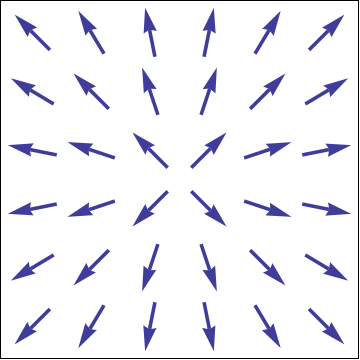## Section1.18Vector Fields

A vector field, $$\FF\text{,}$$ is a function whose output is a vector at each point in the domain of the function. Each such vector should be thought of as "living" (having its tail at) the point at which it is defined, rather than at the origin. A vector field is often written as any of $$\FF(P)\text{,}$$ $$\FF(\rr)\text{,}$$ $$\FF(x,y,z)\text{,}$$ etc., that assigns a vector to each point in the domain, designated by $$P\text{,}$$ $$\rr\text{,}$$ or $$(x,y,z)\text{,}$$ respectively.

Vector fields can be expanded with respect to any basis, so that we can write

\begin{align*} \FF \amp = F_x \,\xhat + F_y \,\yhat + F_z \,\zhat \qquad\hbox{(rectangular)}\\ \amp = F_s \,\shat + F_\phi \,\phat + F_z \,\zhat \qquad\hbox{(cylindrical)}\\ \amp = F_r \,\rhat + F_\theta \,\that + F_\phi \,\phat \qquad\hbox{(spherical)} \end{align*}

where $$F_x$$ is called the component of $$\FF$$ with respect to $$x$$, or simply the $$x$$-component of $$\FF\text{,}$$ and similarly for the other components.  1  More information about the curvilinear basis vectors can be found in Section 1.11. Explicit examples are provided by the polar basis vectors $$\rhat$$ and $$\phat\text{,}$$ which are shown in Figure 1.18.1.Figure 1.18.1. The polar basis vector fields $$\rhat$$ and $$\phat\text{.}$$

You can generate your own examples by editing the Sage code below.

x,y=var('x,y')
p=plot_vector_field((y,x), (x,-10,20), (y,-10,10))
show(p,aspect_ratio=1)

Do not confuse the physicists' notation $$F_x$$ for the $$x$$-component of a vector or vector field with the mathematicians' notation $$f_x$$ for the partial derivative of $$f$$ with respect to $$x\text{.}$$ The distinction will become obvious from the context as you develop experience.